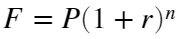# Problem 56105. Compound Interest: Interest Rate

Theorem : Compound Interestwhere,
• F : Future value at the end of n periods
• P : Present value
• r : Annual nominal rate
• n : Total number of compounding periods
Calculate r, given F, P and n.

### Solution Stats

60.0% Correct | 40.0% Incorrect
Last Solution submitted on Nov 23, 2023

### Community Treasure Hunt

Find the treasures in MATLAB Central and discover how the community can help you!

Start Hunting!